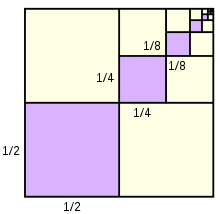# AP members

Insert as many arithmetic sequence members between numbers 1 and 53 that the sum is 702.

x =  24

### Step-by-step explanation:Did you find an error or inaccuracy? Feel free to write us. Thank you!

Tips for related online calculators
Looking for help with calculating arithmetic mean?
Looking for a statistical calculator?

#### Grade of the word problem:

We encourage you to watch this tutorial video on this math problem: